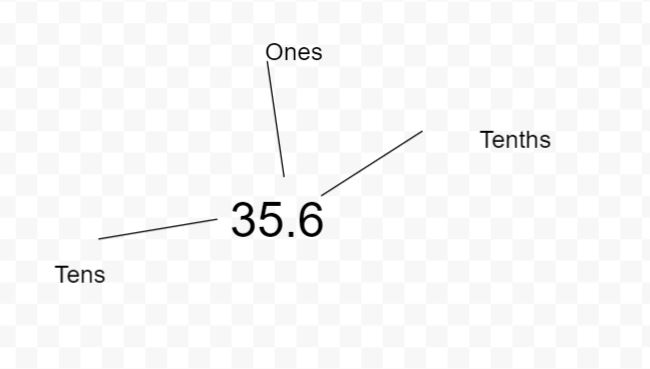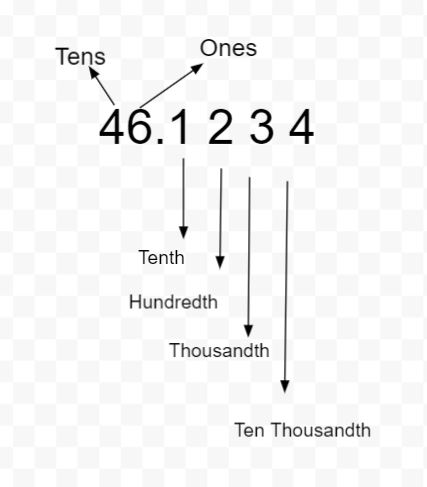###### Latest Topics# Exploring decimal numbers around us

Decimals are a short method of writing fractions and mixed numbers with denominators of powers of ten, such as 10,100,1000, and so on.

Suppose a number has a decimal point. Right after the decimal point, the first digit represents the tenth.

The decimal 0.3, for example, is the fraction 3/10.

To the left of the decimal point is the second digit representing the hundredth.

The decimal 3.26, for example, is the same as the mixed number

3 + 26/100.Place Value: It's all about the location!

Each digit's location (or "place") is critical when writing numbers.

In the case of the number 425:

The "5" is in the One’s position, which means seven ones (seven), the "2" is in the Tens position. The "5" is in the Hundredth position, which means three hundred.

### Components of a decimal number:

The decimal component of the equation

The decimal portion of 15.156, for example, is 156.

The decimal point is a dot (.) that separates the whole from the decimal portion. The decimal point demarcates the whole number portion on the left and the decimal or fractional part on the right.

The spaces on the left side, known as the whole number portion. It starts with ones, then tens, hundreds, thousands, and so on.

The fractional portion or places on the right start with 10. Then, it is followed by hundredths, thousandths, and so on.

A decimal version of the "thirty-five and six-tenths" number is as follows:

Let us take another example of 46.1234.### Finding fraction equivalent of decimal numbers:

The total number of components in 6.006. 6 is the whole number and 6/1000 is the fraction equivalent.

### Using block to explain the concept:

Using base 10 blocks is an excellent method to see decimals.

Consider the case when a big square represents one whole. If the square is split into ten equal-sized pieces, each strip equals one-tenth (0.1). To illustrate hundredths, each strip may be split into 10 smaller squares.

They must know how to write decimal equivalents of a number in tenths and hundredths positions. For example, 2/10 (two divided by hundred) = 0.2 and 7/100 (seven divided hundred) = 0.07.

More examples:

Example 1: What is the value of 3.6?

The whole number component is "3" which is on the left side.

The number 6 is in the "tenths" position, which means "six tenths," or 6/10.

As a result, 3.6 equals "three and three six tenths."

Example 2: What is the value of 15.76?

The entire number portion is "15," which is on the left side.

On the right side, there are two digits: a 7 in the "tenths" position and a 6 in the "hundredths" position.

"13 and 7 tenths and six hundredths" equals 13.76.